# Recursion

For other uses, see Recursion (disambiguation).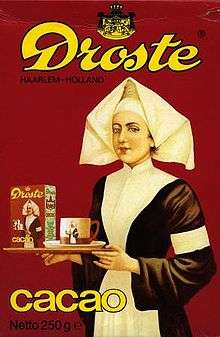A visual form of recursion known as the Droste effect. The woman in this image holds an object that contains a smaller image of her holding an identical object, which in turn contains a smaller image of herself holding an identical object, and so forth. Advertisement for Droste cocoa, c. 1900

Recursion occurs when a thing is defined in terms of itself or of its type. Recursion is used in a variety of disciplines ranging from linguistics to logic. The most common application of recursion is in mathematics and computer science, where a function being defined is applied within its own definition. While this apparently defines an infinite number of instances (function values), it is often done in such a way that no loop or infinite chain of references can occur.

The term is also used more generally to describe a process of repeating objects in a self-similar way. For instance, when the surfaces of two mirrors are exactly parallel with each other, the nested images that occur are a form of infinite recursion.

## Formal definitions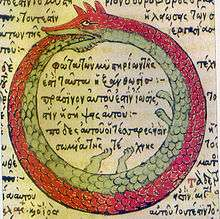Ouroboros, an ancient symbol depicting a serpent or dragon eating its own tail.

In mathematics and computer science, a class of objects or methods exhibit recursive behavior when they can be defined by two properties:

1. A simple base case (or cases)—a terminating scenario that does not use recursion to produce an answer
2. A set of rules that reduce all other cases toward the base case

For example, the following is a recursive definition of a person's ancestors:

• One's parents are one's ancestors (base case).
• The ancestors of one's ancestors are also one's ancestors (recursion step).

The Fibonacci sequence is a classic example of recursion:Many mathematical axioms are based upon recursive rules. For example, the formal definition of the natural numbers by the Peano axioms can be described as: 0 is a natural number, and each natural number has a successor, which is also a natural number. By this base case and recursive rule, one can generate the set of all natural numbers.

Recursively defined mathematical objects include functions, sets, and especially fractals.

There are various more tongue-in-cheek "definitions" of recursion; see recursive humor.

## Informal definitionRecently refreshed sourdough, bubbling through fermentation: the recipe calls for some sourdough left over from the last time the same recipe was made.

Recursion is the process a procedure goes through when one of the steps of the procedure involves invoking the procedure itself. A procedure that goes through recursion is said to be 'recursive'.

To understand recursion, one must recognize the distinction between a procedure and the running of a procedure. A procedure is a set of steps based on a set of rules. The running of a procedure involves actually following the rules and performing the steps. An analogy: a procedure is like a written recipe; running a procedure is like actually preparing the meal.

Recursion is related to, but not the same as, a reference within the specification of a procedure to the execution of some other procedure. For instance, a recipe might refer to cooking vegetables, which is another procedure that in turn requires heating water, and so forth. However, a recursive procedure is where (at least) one of its steps calls for a new instance of the very same procedure, like a sourdough recipe calling for some dough left over from the last time the same recipe was made. This of course immediately creates the possibility of an endless loop; recursion can only be properly used in a definition if the step in question is skipped in certain cases so that the procedure can complete, like a sourdough recipe that also tells you how to get some starter dough in case you've never made it before. Even if properly defined, a recursive procedure is not easy for humans to perform, as it requires distinguishing the new from the old (partially executed) invocation of the procedure; this requires some administration of how far various simultaneous instances of the procedures have progressed. For this reason recursive definitions are very rare in everyday situations. An example could be the following procedure to find a way through a maze. Proceed forward until reaching either an exit or a branching point (a dead end is considered a branching point with 0 branches). If the point reached is an exit, terminate. Otherwise try each branch in turn, using the procedure recursively; if every trial fails by reaching only dead ends, return on the path that led to this branching point and report failure. Whether this actually defines a terminating procedure depends on the nature of the maze: it must not allow loops. In any case, executing the procedure requires carefully recording all currently explored branching points, and which of their branches have already been exhaustively tried.

## In language

Linguist Noam Chomsky among many others has argued that the lack of an upper bound on the number of grammatical sentences in a language, and the lack of an upper bound on grammatical sentence length (beyond practical constraints such as the time available to utter one), can be explained as the consequence of recursion in natural language. This can be understood in terms of a recursive definition of a syntactic category, such as a sentence. A sentence can have a structure in which what follows the verb is another sentence: Dorothy thinks witches are dangerous, in which the sentence witches are dangerous occurs in the larger one. So a sentence can be defined recursively (very roughly) as something with a structure that includes a noun phrase, a verb, and optionally another sentence. This is really just a special case of the mathematical definition of recursion.

This provides a way of understanding the creativity of language—the unbounded number of grammatical sentences—because it immediately predicts that sentences can be of arbitrary length: Dorothy thinks that Toto suspects that Tin Man said that.... Of course, there are many structures apart from sentences that can be defined recursively, and therefore many ways in which a sentence can embed instances of one category inside another. Over the years, languages in general have proved amenable to this kind of analysis.

Recently, however, the generally-accepted idea that recursion is an essential property of human language has been challenged by Daniel Everett on the basis of his claims about the Pirahã language. Andrew Nevins, David Pesetsky and Cilene Rodrigues are among many who that have argued against this. Literary self-reference can in any case be argued to be different in kind from mathematical or logical recursion.

Recursion plays a crucial role not only in syntax, but also in natural language semantics. The word and, for example, can be construed as a function that can apply to sentence meanings to create new sentences, and likewise for noun phrase meanings, verb phrase meanings, and others. It can also apply to intransitive verbs, transitive verbs, or ditransitive verbs. In order to provide a single denotation for it that is suitably flexible, and is typically defined so that it can take any of these different types of meanings as arguments. This can be done by defining it for a simple case in which it combines sentences, and then defining the other cases recursively in terms of the simple one.

### Recursive humor

Recursion is sometimes used humorously in computer science, programming, philosophy, or mathematics textbooks, generally by giving a circular definition or self-reference, in which the putative recursive step does not get closer to a base case, but instead leads to an infinite regress. It is not unusual for such books to include a joke entry in their glossary along the lines of:

Recursion, see Recursion.

A variation is found on page 269 in the index of some editions of Brian Kernighan and Dennis Ritchie's book The C Programming Language; the index entry recursively references itself ("recursion 86, 139, 141, 182, 202, 269"). The earliest version of this joke was in "Software Tools" by Kernighan and Plauger, and also appears in "The UNIX Programming Environment" by Kernighan and Pike. It did not appear in the first edition of The C Programming Language.

Another joke is that "To understand recursion, you must understand recursion." In the English-language version of the Google web search engine, when a search for "recursion" is made, the site suggests "Did you mean: recursion." An alternative form is the following, from Andrew Plotkin: "If you already know what recursion is, just remember the answer. Otherwise, find someone who is standing closer to Douglas Hofstadter than you are; then ask him or her what recursion is."

Recursive acronyms can also be examples of recursive humor. PHP, for example, stands for "PHP Hypertext Preprocessor", WINE stands for "WINE Is Not an Emulator." and GNU stands for "GNU's not Unix".

## In mathematics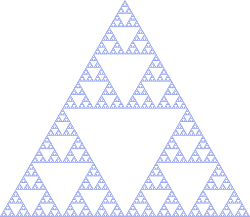The Sierpinski triangle—a confined recursion of triangles that form a fractal

### Recursively defined sets

Main article: Recursive definition

#### Example: the natural numbers

The canonical example of a recursively defined set is given by the natural numbers:

0 is inif n is in, then n + 1 is inThe set of natural numbers is the smallest set satisfying the previous two properties.

#### Example: The set of true reachable propositions

Another interesting example is the set of all "true reachable" propositions in an axiomatic system.

• If a proposition is an axiom, it is a true reachable proposition.
• If a proposition can be obtained from true reachable propositions by means of inference rules, it is a true reachable proposition.
• The set of true reachable propositions is the smallest set of propositions satisfying these conditions.

This set is called 'true reachable propositions' because in non-constructive approaches to the foundations of mathematics, the set of true propositions may be larger than the set recursively constructed from the axioms and rules of inference. See also Gödel's incompleteness theorems.

### Finite subdivision rules

Finite subdivision rules are a geometric form of recursion, which can be used to create fractal-like images. A subdivision rule starts with a collection of polygons labelled by finitely many labels, and then each polygon is subdivided into smaller labelled polygons in a way that depends only on the labels of the original polygon. This process can be iterated. The standard middle thirds' technique for creating the Cantor set is a subdivision rule, as is barycentric subdivision.

### Functional recursion

A function may be partly defined in terms of itself. A familiar example is the Fibonacci number sequence: F(n) = F(n − 1) + F(n − 2). For such a definition to be useful, it must lead to non-recursively defined values, in this case F(0) = 0 and F(1) = 1.

A famous recursive function is the Ackermann function, which—unlike the Fibonacci sequence—cannot easily be expressed without recursion.

### Proofs involving recursive definitions

Applying the standard technique of proof by cases to recursively defined sets or functions, as in the preceding sections, yields structural induction, a powerful generalization of mathematical induction widely used to derive proofs in mathematical logic and computer science.

### Recursive optimization

Dynamic programming is an approach to optimization that restates a multiperiod or multistep optimization problem in recursive form. The key result in dynamic programming is the Bellman equation, which writes the value of the optimization problem at an earlier time (or earlier step) in terms of its value at a later time (or later step).

## In computer science

A common method of simplification is to divide a problem into subproblems of the same type. As a computer programming technique, this is called divide and conquer and is key to the design of many important algorithms. Divide and conquer serves as a top-down approach to problem solving, where problems are solved by solving smaller and smaller instances. A contrary approach is dynamic programming. This approach serves as a bottom-up approach, where problems are solved by solving larger and larger instances, until the desired size is reached.

A classic example of recursion is the definition of the factorial function, given here in C code:

unsigned int factorial(unsigned int n) {
if (n == 0) {
return 1;
} else {
return n * factorial(n - 1);
}
}
`

The function calls itself recursively on a smaller version of the input (n - 1) and multiplies the result of the recursive call by n, until reaching the base case, analogously to the mathematical definition of factorial.

Recursion in computer programming is exemplified when a function is defined in terms of simpler, often smaller versions of itself. The solution to the problem is then devised by combining the solutions obtained from the simpler versions of the problem. One example application of recursion is in parsers for programming languages. The great advantage of recursion is that an infinite set of possible sentences, designs or other data can be defined, parsed or produced by a finite computer program.

Recurrence relations are equations to define one or more sequences recursively. Some specific kinds of recurrence relation can be "solved" to obtain a non-recursive definition.

Use of recursion in an algorithm has both advantages and disadvantages. The main advantage is usually simplicity. The main disadvantage is often that the algorithm may require large amounts of memory if the depth of the recursion is very large.

## In art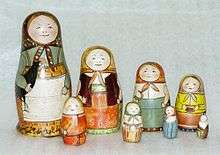Recursive dolls: the original set of Matryoshka dolls by Zvyozdochkin and Malyutin, 1892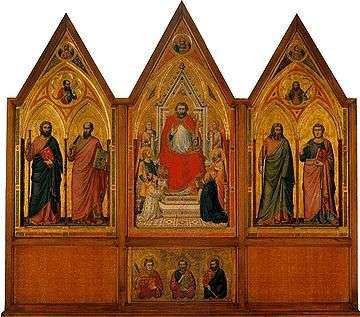Front face of Giotto's Stefaneschi Triptych, 1320, recursively contains an image of itself (held up by the kneeling figure in the central panel).

The Russian Doll or Matryoshka Doll is a physical artistic example of the recursive concept.

Recursion has been used in paintings since Giotto's Stefaneschi Triptych, made in 1320. Its central panel contains the kneeling figure of Cardinal Stefaneschi, holding up the triptych itself as an offering.

M. C. Escher's Print Gallery (1956) is a print which depicts a distorted city which contains a gallery which recursively contains the picture, and so ad infinitum.

## The recursion theorem

In set theory, this is a theorem guaranteeing that recursively defined functions exist. Given a set X, an element a of X and a function, the theorem states that there is a unique function(wheredenotes the set of natural numbers including zero) such thatfor any natural number n.

### Proof of uniqueness

Take two functionsandsuch that:where a is an element of X.

It can be proved by mathematical induction thatfor all natural numbers n:

Base Case:so the equality holds for.
Inductive Step: Supposefor some. ThenHence F(k) = G(k) implies F(k+1) = G(k+1).

By induction,for all.

### Examples

Some common recurrence relations are:

• Golden Ratio:• Factorial:• Fibonacci numbers:• Catalan numbers:,• Computing compound interest
• The Tower of Hanoi
• Ackermann function

1. Pinker, Steven (1994). The Language Instinct. William Morrow.
2. Pinker, Steven; Jackendoff, Ray (2005). "The faculty of language: What's so special about it?". Cognition. 95 (2): 201–236. doi:10.1016/j.cognition.2004.08.004. PMID 15694646.
3. Nevins, Andrew; Pesetsky, David; Rodrigues, Cilene (2009). "Evidence and argumentation: A reply to Everett (2009)" (PDF). Language. 85 (3): 671–681. doi:10.1353/lan.0.0140.
4. Drucker, Thomas (4 January 2008). Perspectives on the History of Mathematical Logic. Springer Science & Business Media. p. 110. ISBN 978-0-8176-4768-1.
5. Barbara Partee and Mats Rooth. 1983. In Rainer Bäuerle et al., Meaning, Use, and Interpretation of Language. Reprinted in Paul Portner and Barbara Partee, eds. 2002. Formal Semantics: The Essential Readings. Blackwell.
6. Hunter, David (2011). Essentials of Discrete Mathematics. Jones and Bartlett. p. 494.
7. Tang, Daisy. "Recursion". Retrieved 24 September 2015. More examples of recursion: Russian Matryoshka dolls. Each doll is made of solid wood or is hollow and contains another Matryoshka doll inside it.
8. "Giotto di Bondone and assistants: Stefaneschi triptych". The Vatican. Retrieved 16 September 2015.
9. Cooper, Jonathan (5 September 2007). "Art and Mathematics". Retrieved 5 September 2015.

## Bibliography

• Dijkstra, Edsger W. (1960). "Recursive Programming". Numerische Mathematik. 2 (1): 312–318. doi:10.1007/BF01386232.
• Johnsonbaugh, Richard (2004). Discrete Mathematics. Prentice Hall. ISBN 0-13-117686-2.
• Hofstadter, Douglas (1999). Gödel, Escher, Bach: an Eternal Golden Braid. Basic Books. ISBN 0-465-02656-7.
• Shoenfield, Joseph R. (2000). Recursion Theory. A K Peters Ltd. ISBN 1-56881-149-7.
• Causey, Robert L. (2001). Logic, Sets, and Recursion. Jones & Bartlett. ISBN 0-7637-1695-2.
• Cori, Rene; Lascar, Daniel; Pelletier, Donald H. (2001). Recursion Theory, Gödel's Theorems, Set Theory, Model Theory. Oxford University Press. ISBN 0-19-850050-5.
• Barwise, Jon; Moss, Lawrence S. (1996). Vicious Circles. Stanford Univ Center for the Study of Language and Information. ISBN 0-19-850050-5. - offers a treatment of corecursion.
• Rosen, Kenneth H. (2002). Discrete Mathematics and Its Applications. McGraw-Hill College. ISBN 0-07-293033-0.
• Cormen, Thomas H., Charles E. Leiserson, Ronald L. Rivest, Clifford Stein (2001). Introduction to Algorithms. Mit Pr. ISBN 0-262-03293-7.
• Kernighan, B.; Ritchie, D. (1988). The C programming Language. Prentice Hall. ISBN 0-13-110362-8.
• Stokey, Nancy,; Robert Lucas; Edward Prescott (1989). Recursive Methods in Economic Dynamics. Harvard University Press. ISBN 0-674-75096-9.
• Hungerford (1980). Algebra. Springer. ISBN 978-0-387-90518-1., first chapter on set theory.Look up recursion or recursivity in Wiktionary, the free dictionary.